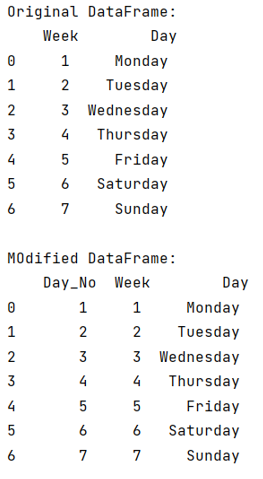# How to Add Incremental Numbers to a New Column Using Pandas?

Given a Pandas DataFrame, we have to add incremental numbers to a new column.
Submitted by Pranit Sharma, on July 17, 2022

Pandas is a special tool that allows us to perform complex manipulations of data effectively and efficiently. Inside pandas, we mostly deal with a dataset in the form of DataFrame. DataFrames are 2-dimensional data structures in pandas. DataFrames consist of rows, columns, and data.

To achieve the task mentioned above, we will first create a DataFrame, and then we need to create a list whose elements lie in a specific range and each value will be incremented by 1.

For creating such kind of list we will use the range() method, which returns elements within the starting value and ending value which will be passed inside it as a parameter.

Then we will insert a new column in DataFrame and assign the newly created list to this column using pandas.DataFrame.insert() method.

To work with pandas, we need to import pandas package first, below is the syntax:

```import pandas as pd
```

Let us understand with the help of an example,

## Python code to add incremental numbers to a new column using Pandas

```# Importing pandas package
import pandas as pd

# Creating a dictionary
d= {
'Week':[1,2,3,4,5,6,7],
'Day':['Monday','Tuesday','Wednesday','Thursday','Friday','Saturday','Sunday']
}

# Creating a DataFrame
df = pd.DataFrame(d)

# Display original DataFrame
print("Original DataFrame:\n",df,"\n")

df.insert(0, 'Day_No', range(1, 1 + len(df)))

# Display modified DataFrame
print("MOdified DataFrame:\n",df)
```

Output:Top MCQs